The process of large eddies becoming smaller is introduced in Sec. 6.6 . The process involves a transfer of kinetic energy from larger to smaller eddies, known as a the energy cascade.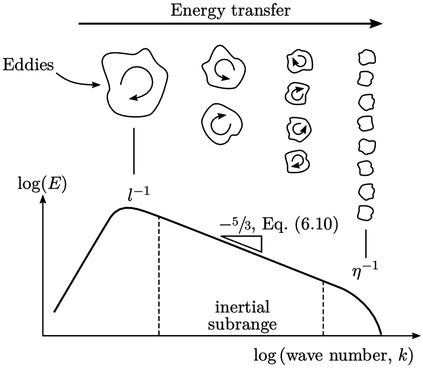The variation in kinetic energy with eddy size is often illustrated by the energy spectrum graph above. The horizontal axis is wave number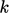,10 which represents the number of eddies per unit length, i.e.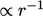where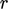= eddy size.

The cascade starts with the largest eddies of sizewith the highest level of turbulent kinetic energy per unit mass (TKE). In the range,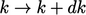, TKE =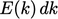.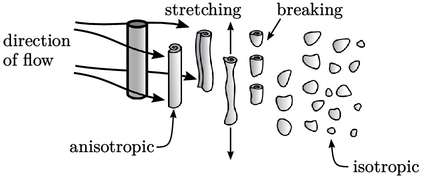Large eddies are usually anisotropic due to the way turbulence is generated. For example, ﬂow past a cylinder causes shedding of vortices whose shape are similar to the cylinder, i.e. longer in the direction of the cylinder axis.

Once moving through open space, eddies quickly stretch, bend, rotate and break, so that quite soon they become “blobs” of vorticity. The turn-over time, initially associated with shedding, decreases with eddy-size — by a factor of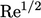at the smallest scales, according to Eq. (6.9 ). Any dominant frequencies, e.g. those due to shedding, are thereby quickly lost.

Kolmogorov’s hypothesis was that the turbulence then becomes isotropic and that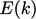is then only a function of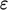and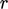when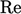is large. From this hypothesis emerges his ﬁve-thirds law for the inertial subrange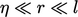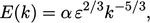(6.10)
where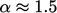for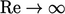. The exponents of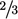and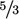are chosen to match the dimensions of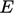which are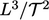, and noting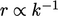.

Experimental data generally supports Eq. (6.10 ) — remarkably, given its simplicity.

10The symbol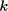, is commonly used in science to denote wave number; it should not be confused with its later use to denote turbulent kinetic energy.

Notes on CFD: General Principles - 6.7 Energy cascade